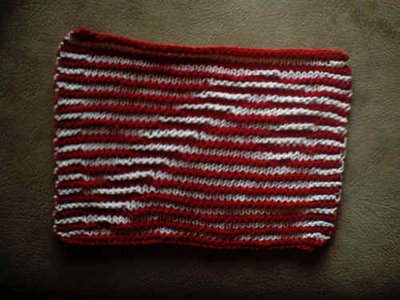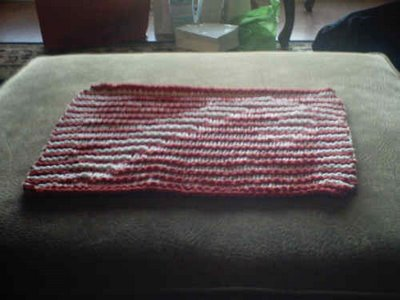## Monday, November 27, 2006

### Free Pattern - Illusion Candy CaneYarn: white (W) & red (R) worsted cotton

Needles: size 5 (illusions look better knitted a little tightly)

CO 41 stitches with red

R1. (R) k across
R2. (R) k across

R3. (W) k across
R4. (W) K3, P35, K3

R5. (R) k across
R6. (R) K3, P35, K3

R7. (W) k across
R8. (W) K3, P35, K3

R9. (R) k across
R10. (R) K5, P2, K34

R11. (W) k across
R12. (W) K3, P2, K2, P31,K3

R13. (R) k across
R14. (R) K3, P7, K31

R15. (W) k across
R16. (W) K10, P28, K3

R17. (R) k across
R18. (R) K5, P7, K29

R19. (W) k across
R20. (W) K3, P2, K7, P26,K3

R21. (R) k across
R22. (R) K7, P7, K27

R23. (W) k across
R24. (W) K3, P4, K7, P24,K3

R25. (R) k across
R26. (R) K9, P7, K25

R27. (W) k across
R28. (W) K3, P6, K7, P22,K3

R29. (R) k across
R30. (R) K11, P7, K13, P3, K7

R31. (W) k across
R32. (W) K3, P8, K7, P13,K3, P4, K3

R33. (R) k across
R34. (R) K13, P7, K9, P7, K5

R35. (W) k across
R36. (W) K3, P10, K7, P9, K7, P2, K3

R37. (R) k across
R38. (R) K15, P7, K9, P6, K4

R39. (W) k across
R40. (W) K3, P12, K7, P9, K6, P1, K3

R41. (R) k across
R42. (R) K18, P7, K7, P6, K3

R43. (W) k across
R44. (W) K3, P15, K7, P7, K9

R45. (R) k across
R46. (R) K20, P7, K3, P7, K4

R47. (W) k across
R48. (W) K3, P17, K7, P3, K7, P1, K3

R49. (R) k across
R50. (R) K22, P13, K6

R51. (W) k across
R52. (W) K3, P19, K13, P3, K3

R53. (R) k across
R54. (R) K27, P4, K10

R55. (W) k across
R56. (W) K3, P24, K4, P7, K3

R57. (R) k across
R58. (R) K3, P35, K3

R59. (W) k across
R60. (W) K3, P35, K3

R61. (R) k across
R62. (R) K3, P35, K3

R63. (W) k across
R64. (W) K3, P35, K3

R65. (R) k across
R66. (R) k across

Bind off.

Weave in ends.This work is licensed under a
Creative Commons Attribution-Share Alike 3.0 Unported License.

#### 2 comments:Anonymous said...

How does one change to another color on your illusion dishcloths? Do you just tie on at the beginning of the row or what? Thanks.Anonymous said...

sim;py carry unused color yarn up the side, it blends in and you don't have knots every other row